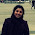Latest Articles

# All from 9 and the last from 10 ...continued

Info Post
CASE 1: When both the multipliers are lower than the base
Example :
95 * 97 =?
We see from above that both numbers are less than 100 (nearest base).To get these numbers we have to subtract 5 for 95 from 100 and 3 for 97 from 100.
So,
95 (-5)
97 (-3)
(95-3) or(97 - 5) | (5 *3)
=92 | 15
=9215
Therefore,
Result of 95 * 97 =9215

CASE 2: When both the multipliers are higher than the base
Example :
103 * 109 =?
The above two numbers are greater than 100.To get these numbers we have to add 3 for 103 from 100 and 9 for 109 from 100.
So,
103 (+3)
109 (+9)
-----------
(103+9) or (109+3) | ( 9 *3)
=112 | 27
=11227
Therefore,
Result =103 * 109 =11227

CASE 3:When one number is more and the other less than the base
Example :
98 * 103 = ?
Now we see that 98 is 2 less than 100 and 105 is 5 more than 100.
So,
98 (-2)
103 (3)
-----------
(98+3) or (103 -2) | (3*-2)
=101 | (-6) ....here we got -6 ,so subtract this from 100 and subtract one from 101 to get our final result.
=(101-1) | (100-6)
= 100 |94
=10094
Therefore ,
Result =98 * 103 =10094

Another Example from all the Three Cases :

 CASE 1: 89 * 91 =? Subtract from 100, 89 (-11) 91 (-9) -------- (89-9) or (91-11) | 11 * 9 =80|99 =8099 Result :89 * 91 =8099 CASE 2: 112 * 101=? Add from 100, 112 (+12) 101 (+1) ---------- (112+1) or (101+12) | 12*1 =113 |12 =11312 Result : 112 * 101 =11312 CASE 3: 105 * 91 =? ...Here, 105 (+5) 91 (-9) ----------- (105-9) or (91 + 5) | (5 * -9) = 96 | (-45) =(96 - 1) | (100-45) as stated from above =95 | 55 =9555 Result : 105 * 91 = 9555
Share:

1.Hi. I just saw your posts. They are very useful. I knew few of the vedic maths tricks. But these one are new for me. Please keep writing. :)
Thank you.

1.Thank you Deepika ! Yes, I will write whenever I can :)

2.Thank you sister .. this post help me understand nikhilam ..

Thank You for visiting Momscribe.com !